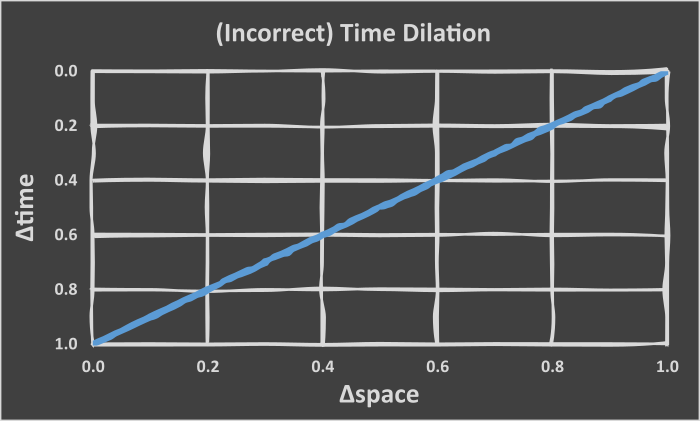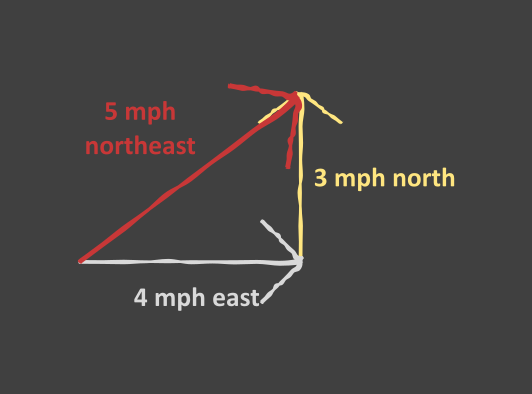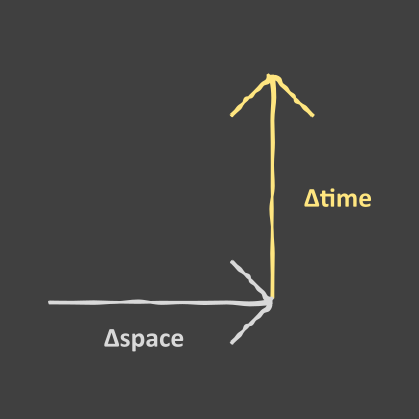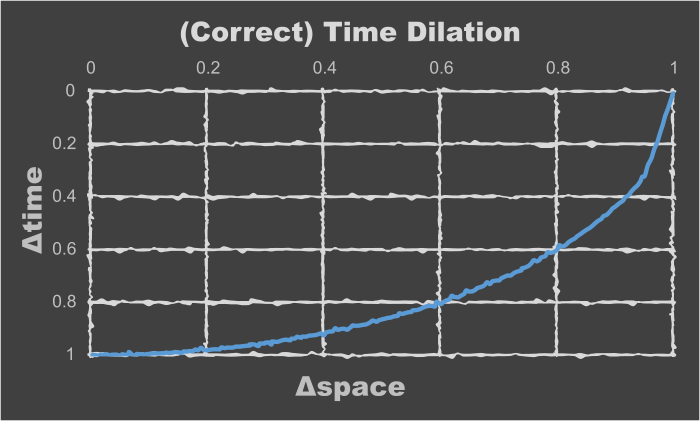Time as distance. We live in three dimensions spatially. Add time, and now we have four. But is time really that different than space?

Our language - or at least English - even allows using the same adjectives and adverbs to describe both time and distance. It was a long time ago. Anacondas are long snakes. I will be home shortly. Someone under four feet tall is short.

We can’t, however, quantitatively measure time and space using the same units. That is, we don’t measure time in feet, meters, miles, or kilometers. We also don’t measure distance in seconds, hours, days, or years.

Or can we? Is there some way to convert spatial dimensions into time dimensions - and vice versa?

I think too much, but I’m not the first person to ask this question.

This thought I had that a certain length of time is equal to a certain length of space also coincides with the idea that everything is traveling at the same speed… Some things, like us, have all their speed in the time dimension. Other things, like light, have all their speed in the spatial dimension.

So, if you want to increase your speed in a spatial dimension, you’ll have to decrease your speed in the time dimension.

Back to my idea (well, someone else’s idea, really) that time can be converted to distance. Since we have something that has all its speed in the spatial dimension - namely, light - that might be our conversion.

So, the speed of light is 299,792,458 meters per second. That means our conversion is 299,792,458 meters equals one second.

In other words, just like 1,000 meters equals 1 kilometer, or 5,280 feet equals 1 mile, 299,792,458 meters equals one second. Time and distance are measuring the same thing, we just perceive them differently.

Back to the “always going the same speed” idea: my first thought is that your speed vector and time vector would combine:Time and space vectors combining in the same direction.

The equation would look something like:

``````Δspace + Δtime = total speed
``````

Where “total speed” would equal the speed of light (aka “max speed”) and Δspace and Δtime would each be a fraction of that max speed. To make things easy, we’ll use the traditional “c” variable for the “total speed”:

``````Δspace + Δtime = c
``````

where

``````0 < Δspace < c
0 < Δtime < c
``````

This would make the time dilation equation linear:

``````Δtime = c - Δspace
``````Incorrect time dilation graph.

It turns out there are many people much smarter than I am, and the equation for time dilation actually is:

``````t = t₀/(1-v²/c²)¹ᐟ²
``````

To clarify that ¹ᐟ² is the square root:

``````t = t₀/√(1-v²/c²)
``````

or

``````t² = t₀²/(1-v²/c²)
``````

My original hypothesis didn’t make much sense, anyway. If I’m traveling on Earth such that my eastern velocity is 4 mph and my northern velocity is 3 mph, I’m not traveling 8 mph in a northeast direction. Instead, I’m traveling 5 mph in a north-east direction:Northeast travel doesn’t combine linearly.

We can use the good ole Pythagorean theorem to figure that out:

``````a² + b² = c²
4² + 3² = 16 + 9 = 25
c² = 25; c = 5
``````

Now let’s try it with time and space:Time and space vectors combining orthogonally.

``````Δspace² + Δtime² = c²
Δtime² = c² - Δspace²
Δtime = √(c² - Δspace²)
``````

That’s closer to the correct equation:

``````t = t₀/√(1-v²/c²)
``````

I’m dealing with square roots now, but I only have one time variable (Δtime) where the correct equation has two (t and t₀). Also, the correct equation’s space variable (the v²) is divided by the speed of light squared (the c²).

This is rectified by stating everything as a fraction of the speed of light. This makes c=1, thus eliminating the “divided by the speed of light squared” part:

``````t = t₀/√(1-v²/c²)
t = t₀/√(1-v²/1²)
t = t₀/√(1-v²/1)
t = t₀/√(1-v²)
``````

Either t or t₀ represents a whole c, where the other is a percentage c. That is, one of them is 1, while the other is between 0 and 1 (inclusive). I’m going to assign t to 1, since that will make my equation the same as the correct one:

``````t = t₀/√(1-v²)
1 = t₀/√(1-v²)
√(1-v²) = t₀
t₀ = √(1-v²)
``````

Remember, my equation was:

``````Δtime = √(c² - Δspace²)
``````

Allowing Δtime and Δspace to be fractions of c (i.e. from 0 to 1, inclusive), as well as c itself (i.e. set c to 1), my equation becomes:

``````Δtime = √(1² - Δspace²)
Δtime = √(1 - Δspace²)
``````Correct time dilation graph.

(Assigning c to one, as well as assigning several other physical constants to one, has been done before by people much smarter than me. See natural units on Wikipedia.)